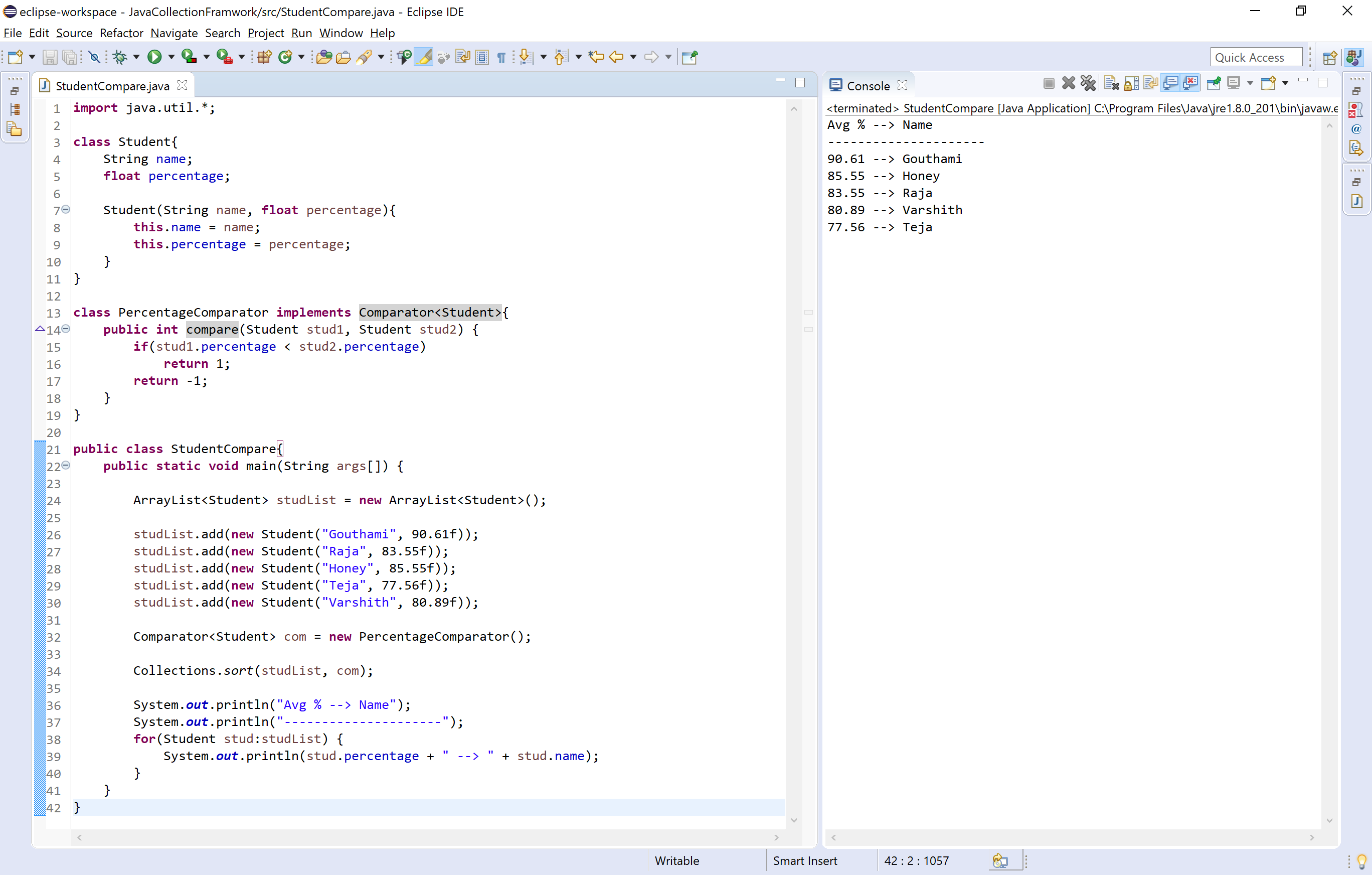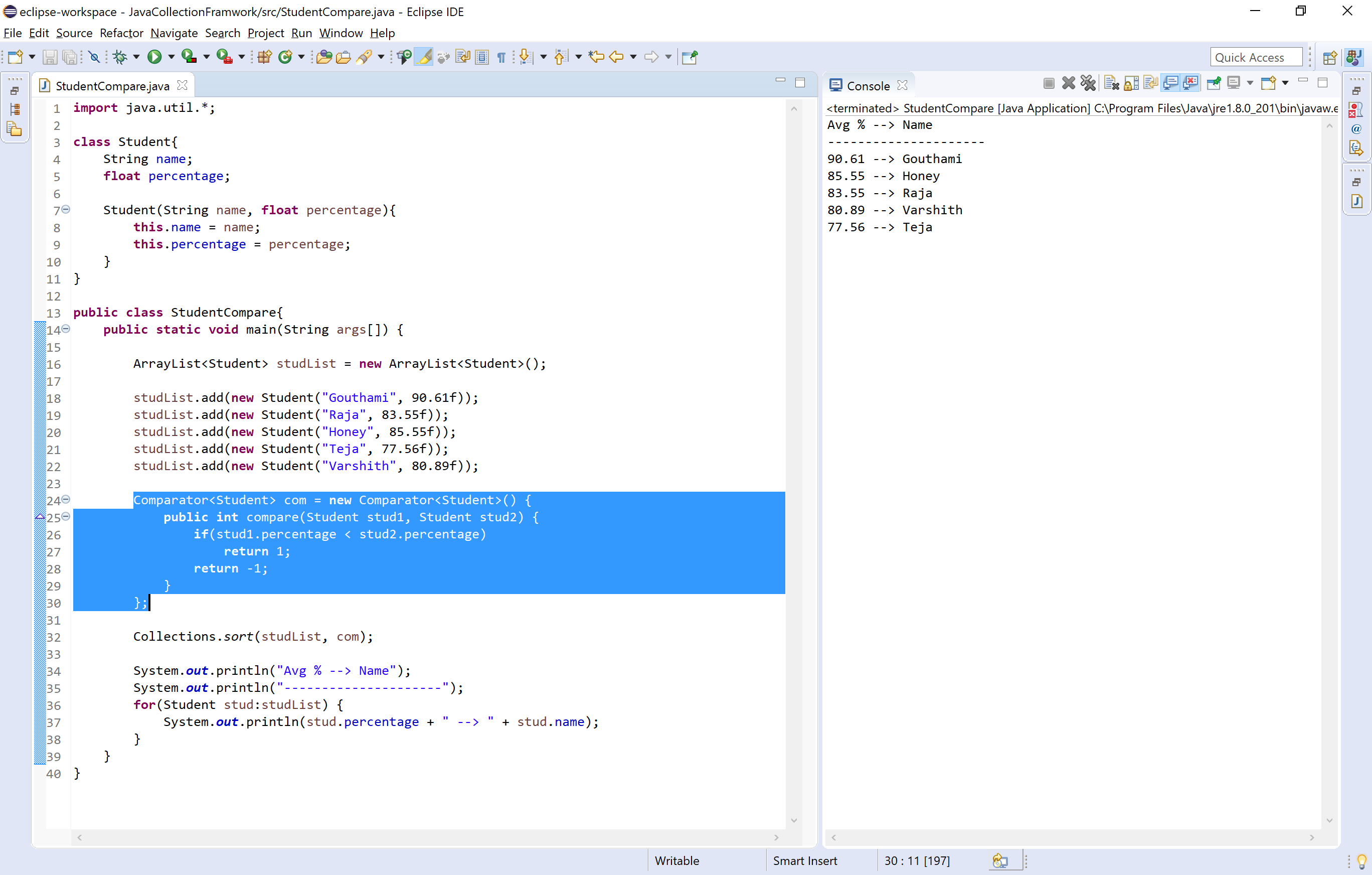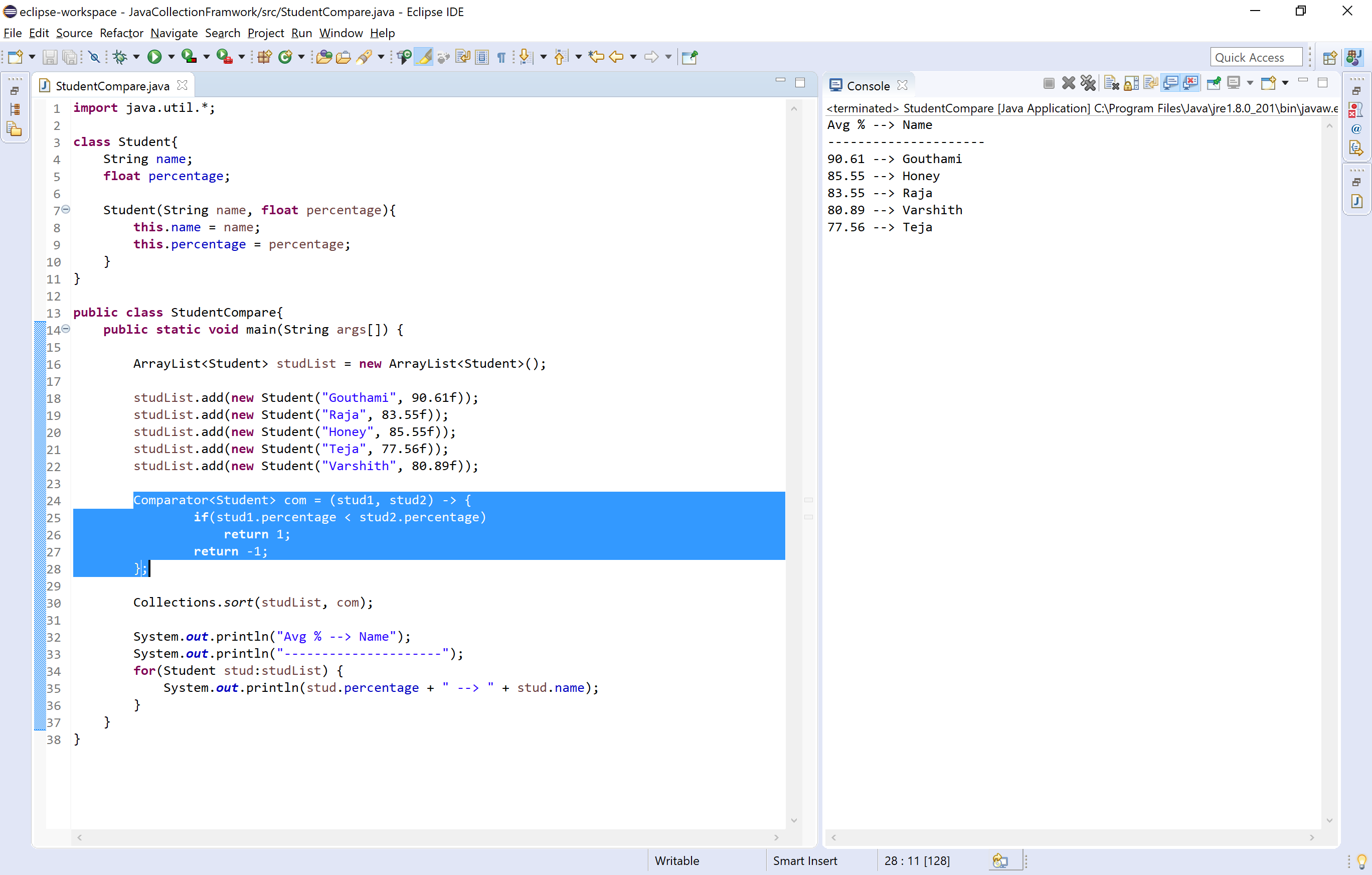The perfect place for easy learning...

×

# Comparators in java

The Comparator is an interface available in the java.util package. The java Comparator is used to order the objects of user-defined classes. The java Comparator can compare two objects from two different classes.

Using the java Comparator, we can sort the elements based on data members of a class. For example, we can sort based on roolNo, age, salary, marks, etc.

The Comparator interface has the following methods.

Method Description
int compare(Object obj1, Object obj2) It is used to compares the obj1 with o bj2 .
boolean equals(Object obj) It is used to check the equity between current object and argumented object.

The Comparator can be used in the following three ways.

• Using a seperate class that implements Comparator interface.
• Using anonymous class.
• Using lamda expression.

## Using a seperate class

We use the following steps to use Comparator with a seperate class.

• Step - 1: Create the user-defined class.
• Step - 2: Create a class that implements Comparator interface.
• Step - 3: Implement the comapare( ) method of Comparator interface inside the above defined class(step - 2).
• Step - 4: Create the actual class where we use the Compatator object with sort method of Collections class.
• Step - 5: Create the object of Compatator interface using the class crearted in step - 2.
• Step - 6: Call the sort method of Collections class by passing the object created in step - 6.
• Step - 7: Use a for-each (any loop) to print the sorted information.

Let's consider an example program to illustrate Comparator using a separate class.

Example
``````import java.util.*;

class Student{

String name;
float percentage;

Student(String name, float percentage){
this.name = name;
this.percentage = percentage;
}

}

class PercentageComparator implements Comparator<Student>{
public int compare(Student stud1, Student stud2) {
if(stud1.percentage < stud2.percentage)
return 1;
return -1;
}
}

public class StudentCompare{

public static void main(String args[]) {

ArrayList<Student> studList = new ArrayList<Student>();

Comparator<Student> com = new PercentageComparator();

Collections.sort(studList, com);

System.out.println("Avg % --> Name");
System.out.println("---------------------");
for(Student stud:studList) {
System.out.println(stud.percentage + " --> " + stud.name);
}
}
}```
```## Using anonymous class

We use the following steps to use Comparator with anonymous class.

• Step - 1: Create the user-defined class.
• Step - 2: Create the actual class where we use the Compatator object with sort method of Collections class.
• Step - 3: Create the object of Compatator interface using anonymous class and implement compare method of Comparator interface.
• Step - 4: Call the sort method of Collections class by passing the object created in step - 6.
• Step - 5: Use a for-each (any loop) to print the sorted information.

Let's consider an example program to illustrate Comparator using a separate class.

Example
``````import java.util.*;

class Student{

String name;
float percentage;

Student(String name, float percentage){
this.name = name;
this.percentage = percentage;
}

}

public class StudentCompare{

public static void main(String args[]) {

ArrayList<Student> studList = new ArrayList<Student>();

Comparator<Student> com = new Comparator<Student>() {
public int compare(Student stud1, Student stud2) {
if(stud1.percentage < stud2.percentage)
return 1;
return -1;
}
};

Collections.sort(studList, com);

System.out.println("Avg % --> Name");
System.out.println("---------------------");
for(Student stud:studList) {
System.out.println(stud.percentage + " --> " + stud.name);
}
}
}```
```## Using lamda expression

We use the following steps to use Comparator with lamda expression.

• Step - 1: Create the user-defined class.
• Step - 2: Create the actual class where we use the Compatator object with sort method of Collections class.
• Step - 3: Create the object of Compatator interface using lamda expression and implement the code for compare method of Comparator interface.
• Step - 4: Call the sort method of Collections class by passing the object created in step - 6.
• Step - 5: Use a for-each (any loop) to print the sorted information.

Let's consider an example program to illustrate Comparator using a separate class.

Example
``````import java.util.*;

class Student{

String name;
float percentage;

Student(String name, float percentage){
this.name = name;
this.percentage = percentage;
}
}

public class StudentCompare{

public static void main(String args[]) {

ArrayList<Student> studList = new ArrayList<Student>();

Comparator<Student> com = (stud1, stud2) -> {
if(stud1.percentage < stud2.percentage)
return 1;
return -1;
};

Collections.sort(studList, com);

System.out.println("Avg % --> Name");
System.out.println("---------------------");
for(Student stud:studList) {
System.out.println(stud.percentage + " --> " + stud.name);
}
}
}```
```